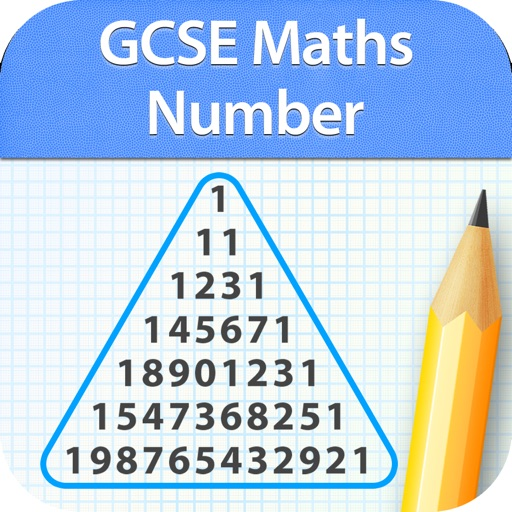## Here comes the most comprehensive Number app with over 750 questions and 76 revision to revise from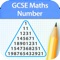# GCSE Maths : Number Revision

by GCSE Exams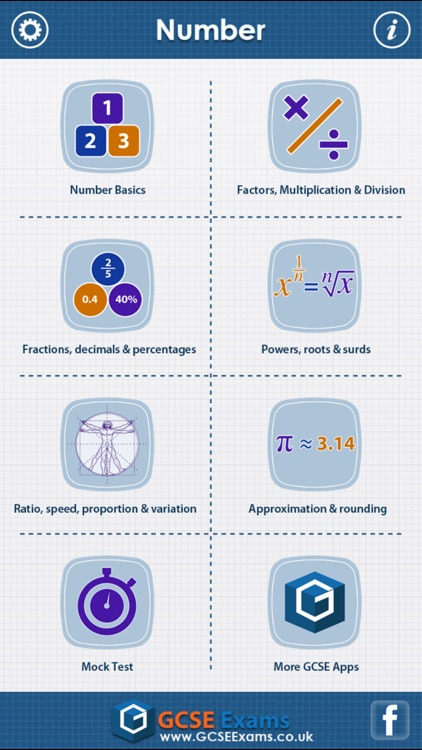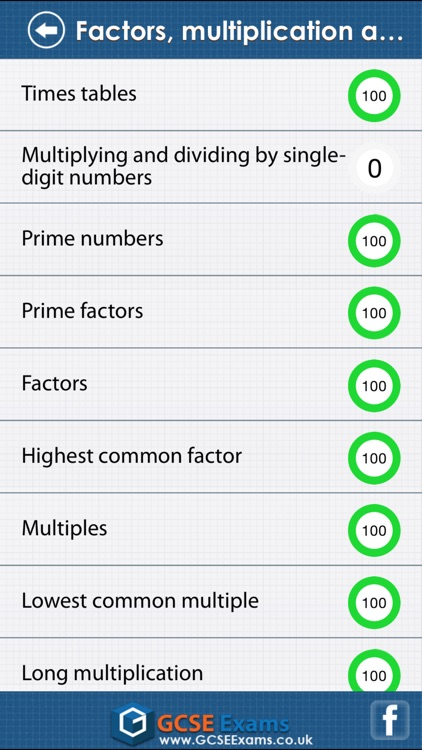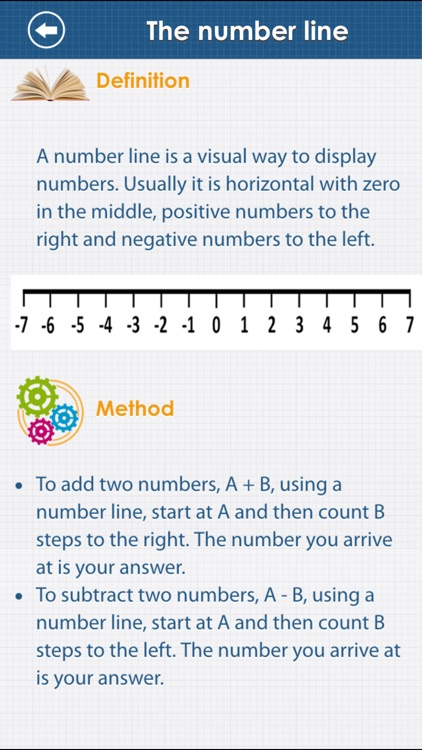Here comes the most comprehensive Number app with over 750 questions and 76 revision to revise from.### App Details

Version
2.0
Rating
NA
Size
28Mb
Genre
Education Reference
Last updated
June 20, 2017
Release date
September 11, 2012

### App Screenshots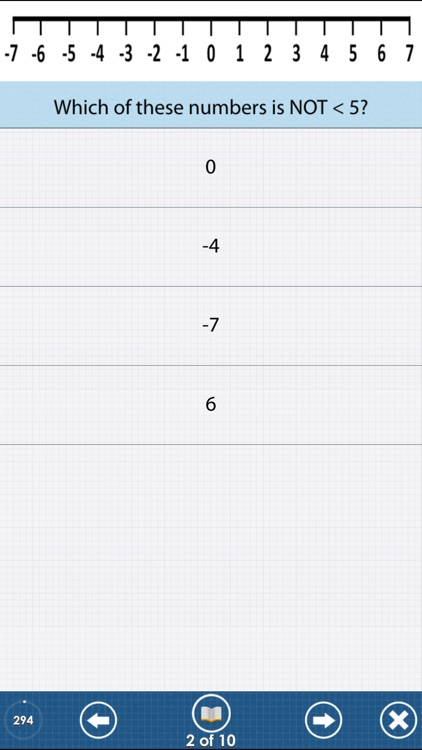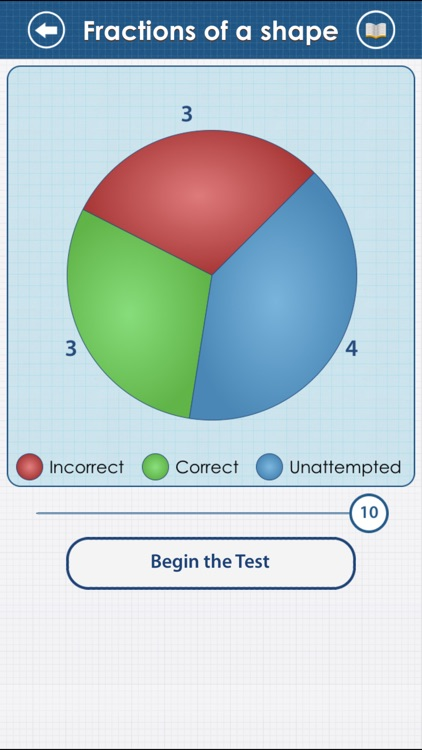### App Store Description

Here comes the most comprehensive Number app with over 750 questions and 76 revision to revise from.
Includes syllabus covered by all exams boards including OCR, EDEXCEL,AQA,WJEC,CCEA  etc.

Features include:

HIGHEST QUALITY and QUANTITY
760 questions and 76 revision notes in all just for Number!. High quality content written by experienced GCSE tutors.

REVISE BY TOPIC
Basics, factors, multiplication, division, decimals, fractions, percentages, powers, roots and surds, ratios, speed, proportion and variation, approximation and rounding.

MOCK TEST
Mixed questions from all topics.

INSTANT FEEDBACK

REVIEW WITH EXPLANATION
Review each question at the end of the test. Know the right answer with detailed explanation for each question.

PROGRESS METER
With our unique progress tracking feature including pie charts and bar graphs showing your progress, you know you are ready to take on the real test at the board when your progress meter says 100%.

More details on topics:

1. NUMBER BASICS

The number line (F)
Ordering Numbers and Place Values (F)
Negative Numbers (F)
Addition and subtraction with negative numbers (F)
Order of operations and BODMAS (F)
Number problems in words (F)

2. FACTORS, MULTIPLICATIONS AND DIVISION

Times tables (F)
Multiplying and dividing by single-digit numbers (F)
Prime numbers (F)
Prime factors (F)
Factors (F)
Highest common factor (F)
Multiples (F)
Lowest common multiple (F)
Long multiplication (F)
Long division (F)
Multiplying and dividing with negative numbers (F)
Multiplying and dividing by decimals (F)
Real-life problems using multiplication and division (F)

3. FRACTION, DECIMALS AND PERCENTAGES

Fractions of a shape (F)
Finding a fraction of a quantity (F)
Improper fractions and mixed numbers (F)
Equivalent fractions (F)
One quantity as a fraction of another (F)
Converting fractions into decimals (F)
Converting decimals into fractions (F)
Multiplying fractions (F)
Dividing fractions (F)
Reciprocals (F)
Fraction problems in words (F)
Decimals - multiplication and division (F)
Equivalent percentages, fractions and decimals (F)
Calculating a percentage of a quantity (F)
One quantity as a percentage of another (F)
Percentage change (F)
Percentage problems in words (F)
Compound interest (H)
Reverse percentage (H)

4. POWERS, ROOTS AND SURDS

Square numbers (F)
Powers of 10 (F)
Multiplying using powers of 10 (F)
Dividing using powers of 10 (F)
Square roots and cube roots (F)
Multiplying powers (F)
Dividing powers (F)
Calculated Powers (F)
Power of a power (F)
Fractional and zero powers (H)
Negative powers (H)
Standard form (H)
Surds and pi (H)
Rationalising the denominator (H)
Solving problems with surds (H)

5. RATIO, SPEED, PROPORTION AND VARIATION

Dividing amounts in ratios (F)
Ratios and fractions (F)
Calculating with ratios (F)
Ratio problems in words (F)
Speed (F)
Density (H)
Direct proportion (H)
Inverse proportion (H)

6. APPROXIMATION and ROUNDING

Significant figures (F)
Approximation of calculations (F)
Limits of accuracy (F)
Calculating with limits of accuracy (F)
Decimal places (F)
Rounding (F)

Disclaimer:
AppAdvice does not own this application and only provides images and links contained in the iTunes Search API, to help our users find the best apps to download. If you are the developer of this app and would like your information removed, please send a request to takedown@appadvice.com and your information will be removed.